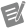# Waveform Channels

Edition Date: June 2018
Part Number: 370858P-01
View Product InfoDIAdem 2017 HelpDIAdem 2018 HelpDIAdem 2019 HelpDIAdem 2020 HelpDIAdem 2020 SP1 Help

Waveform channels contain the x-part and the y-part of a curve in a channel. The x-part might be the measured time, for example. The Waveform properties specify the x-part that is a linear specification with a start value and a step width. The y-part contains the measurement values.

To play waveform channels, the property Waveform x-unit must be a time unit and the property Waveform x-stepwidth must be greater than 5.208333 µs. The channel must be completely accessible in the computer memory and not just registered.

Use the function Numeric channels <-> Waveform channels to convert waveform channels into numeric channels and vice versa. When you display waveform channels in axis systems, you must not specify an x-channel. You specify the waveform channels as the y-channels. If you do specify an x-channel, DIAdem interprets the waveform channels as numeric channels.

In the Data Portal you can play waveform channels acoustically. Click the loudspeaker symbol at the top on the right in the channel preview to enable the sound and then select a waveform channel in the structure view or the list view of the Data Portal. DIAdem plays the waveform channel once. To play waveform channels, the property Waveform x-unit must be a time unit and the property Waveform x-stepwidth must be greater than 5.208333 µs. The channel must be completely accessible in the computer memory and not just registered.

The time values of a waveform channel are relative to the absolute start time of a measurement. This means that DIAdem calculates and displays waveform channels with a relative time reference. However, DIAdem does not include the waveform property wf_start_time.

When DIAdem calculates quantity-based with waveform channels, DIAdem uses the waveform x-unit as the unit of the x-channel that is not specified, and uses the unit of the waveform channel as the y-unit. If, for example, you differentiate a waveform channel that has the unit m and the waveform x-unit s, the result channel has the unit m/s.Note  If you, for example, delete or add channel values, DIAdem does not automatically adjust the waveform properties wf_start_time, wf_start_offset, and wf_increment. Adjust the waveform properties manually either with a script or in the properties window in order to retain the time reference between the x-part and the y-part of the waveform channel.

## Rules for Calculating with Waveform Channels

If you calculate with waveform channels, observe the following rules:

1. Mathematical functions that do not support waveform channels use waveform channels like numeric channels.

2. Mathematical functions that support waveform channels return waveform channels as a result if at least one input channel is a waveform channel. These mathematical functions follow one or more of the following rules.

2.1 If the mathematical function requires an x-input channel (time channel) and a y-input channel (signal channel), do not specify an x-input channel and specify a waveform channel as the y-input channel. The function returns one or more waveform channels as the result.

2.2 If the mathematical function requires an x-input channel (time channel) and one or more y-input channels (signal channels), do not specify an x-input channel. All y-input channels must be waveform channels that have the same x-parts.

2.3 If the mathematical function needs an input channel, this input channel must be a waveform channel so that the function can return a waveform channel as the result channel. If the mathematical function requires several input channels, at least one input channel must be a waveform channel and all the waveform input channels that are involved must have the same x-parts to ensure that the function returns a waveform channel as the result channel. The x-parts coincide when the properties Waveform x-offset, Waveform x-step width, and in quantity based calculations also the property Waveform x-unit are the same. The waveform result channel takes on all the waveform properties of the first waveform input channel.

## Examples

The following example calculates the linear regression for the Group1/Input1 waveform channel. You do not specify an x-channel and the command returns the y-waveform channel Regress_Y according to rule 2.1.

`Call ChnRegrXYCalc("","Group1/Input1","","/Regress_Y","linear","Partition complete area",100,1)`

The following example integrates the signal saved in the Group1/Input1 waveform channel. You do not specify an x-channel. The command returns the waveform channel Y_Integral according to the rules 2.2 and 2.3.

`Call ChnIntegrate("","Group1/Input1","/Y_Integral")`

The following example calculates the arithmetic mean for two waveform channels rowwise and returns the new waveform Result channel with the x-part of the first input channel Group1/Input1 according to rule 2.3.

`Call ChnAverage("'Group1/Input1' - 'Group1/Input2'","/Result")`

The following example maps the signal saved in the Group1/Input2 waveform channel on the x-value range of the signal saved in the Group1/Input1 waveform channel.

```Dim XChannel
XChannel = ChnFromWfXGen("Group1/Input1")
Call ChnMapLinCalc("","Group1/Input2",XChannel,"/Input2_mapped",1,"const. value",NOVALUE,"analog")
Call ChnToWfChn(XChannel,"/Input2_mapped",1)```